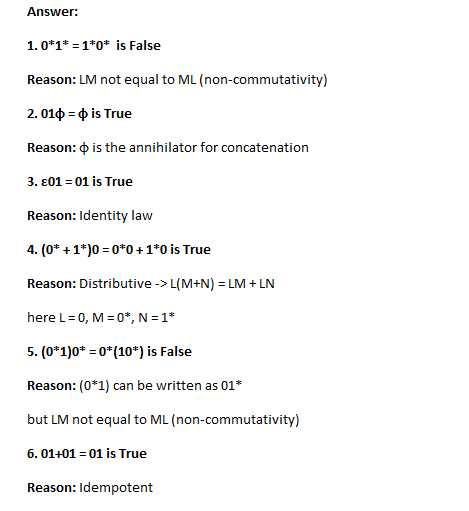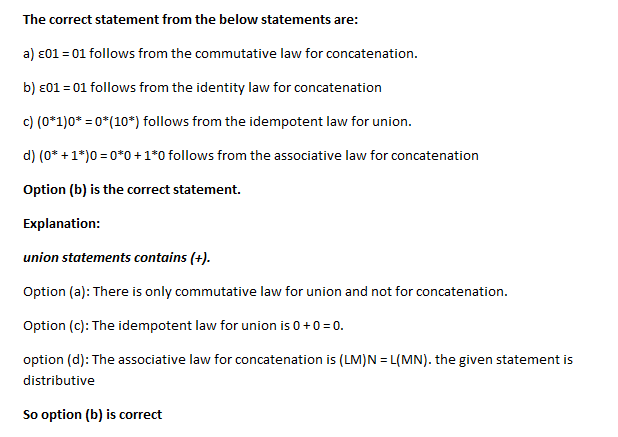# Answered! Consider the truth or falsehood of six equivalences for regular expressions. If the equivalence is true, you must also…

Consider the truth or falsehood of six equivalences for regular expressions. If the equivalence is true, you must also identify the law from which it follows. In each case the statement R = S is conventional shorthand for “L(R) = L(S).” The six proposed equivalences are:

1. 0*1* = 1*0*

Don't use plagiarized sources. Get Your Custom Essay on
Answered! Consider the truth or falsehood of six equivalences for regular expressions. If the equivalence is true, you must also…
GET AN ESSAY WRITTEN FOR YOU FROM AS LOW AS \$13/PAGE

2. 01φ = φ

3. ε01 = 01

4. (0* + 1*)0 = 0*0 + 1*0

5. (0*1)0* = 0*(10*)

6. 01+01 = 01

Identify the correct statement from the list below.

Note: we use φ for the empty set, because the correct symbol is not recognized by Internet Explorer.

a) ε01 = 01 follows from the commutative law for concatenation.

b) ε01 = 01 follows from the identity law for concatenation

c) (0*1)0* = 0*(10*) follows from the idempotent law for union.

d) (0* + 1*)0 = 0*0 + 1*0 follows from the associative law for concatenation